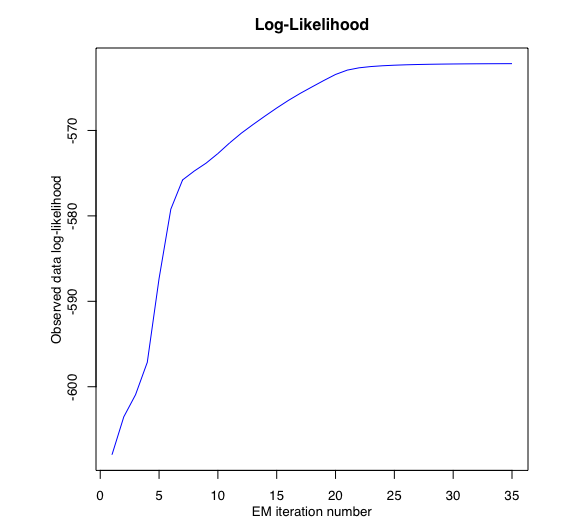# Introduction

TMoE (t Mixture-of-Experts) provides a flexible and robust modelling framework for heterogenous data with possibly heavy-tailed distributions and corrupted by atypical observations. TMoE consists of a mixture of K t expert regressors network (of degree p) gated by a softmax gating network (of degree q) and is represented by:

• The gating network parameters alpha’s of the softmax net.
• The experts network parameters: The location parameters (regression coefficients) beta’s, scale parameters sigma’s, and the degree of freedom (robustness) parameters nu’s. TMoE thus generalises mixtures of (normal, t, and) distributions and mixtures of regressions with these distributions. For example, when $$q=0$$, we retrieve mixtures of (t-, or normal) regressions, and when both $$p=0$$ and $$q=0$$, it is a mixture of (t-, or normal) distributions. It also reduces to the standard (normal, t) distribution when we only use a single expert ($$K=1$$).

Model estimation/learning is performed by a dedicated expectation conditional maximization (ECM) algorithm by maximizing the observed data log-likelihood. We provide simulated examples to illustrate the use of the model in model-based clustering of heterogeneous regression data and in fitting non-linear regression functions.

It was written in R Markdown, using the knitr package for production.

See help(package="meteorits") for further details and references provided by citation("meteorits").

# Application to a simulated dataset

## Generate sample

n <- 500 # Size of the sample
alphak <- matrix(c(0, 8), ncol = 1) # Parameters of the gating network
betak <- matrix(c(0, -2.5, 0, 2.5), ncol = 2) # Regression coefficients of the experts
sigmak <- c(0.5, 0.5) # Standard deviations of the experts
nuk <- c(5, 7) # Degrees of freedom of the experts network t densities
x <- seq.int(from = -1, to = 1, length.out = n) # Inputs (predictors)

# Generate sample of size n
sample <- sampleUnivTMoE(alphak = alphak, betak = betak, sigmak = sigmak,
nuk = nuk, x = x)
y <- sample$y ## Set up tMoE model parameters K <- 2 # Number of regressors/experts p <- 1 # Order of the polynomial regression (regressors/experts) q <- 1 # Order of the logistic regression (gating network) ## Set up EM parameters n_tries <- 1 max_iter <- 1500 threshold <- 1e-5 verbose <- TRUE verbose_IRLS <- FALSE ## Estimation tmoe <- emTMoE(X = x, Y = y, K, p, q, n_tries, max_iter, threshold, verbose, verbose_IRLS) ## EM - tMoE: Iteration: 1 | log-likelihood: -529.945288794032 ## EM - tMoE: Iteration: 2 | log-likelihood: -527.348101613744 ## EM - tMoE: Iteration: 3 | log-likelihood: -526.697258494959 ## EM - tMoE: Iteration: 4 | log-likelihood: -526.100920519297 ## EM - tMoE: Iteration: 5 | log-likelihood: -525.530707141445 ## EM - tMoE: Iteration: 6 | log-likelihood: -525.004975573049 ## EM - tMoE: Iteration: 7 | log-likelihood: -524.537304371765 ## EM - tMoE: Iteration: 8 | log-likelihood: -524.134230296182 ## EM - tMoE: Iteration: 9 | log-likelihood: -523.796173749721 ## EM - tMoE: Iteration: 10 | log-likelihood: -523.519134936736 ## EM - tMoE: Iteration: 11 | log-likelihood: -523.296473104273 ## EM - tMoE: Iteration: 12 | log-likelihood: -523.120395143099 ## EM - tMoE: Iteration: 13 | log-likelihood: -522.983018797515 ## EM - tMoE: Iteration: 14 | log-likelihood: -522.877027662562 ## EM - tMoE: Iteration: 15 | log-likelihood: -522.796003916234 ## EM - tMoE: Iteration: 16 | log-likelihood: -522.734538519799 ## EM - tMoE: Iteration: 17 | log-likelihood: -522.68820514474 ## EM - tMoE: Iteration: 18 | log-likelihood: -522.653461853027 ## EM - tMoE: Iteration: 19 | log-likelihood: -522.627523155938 ## EM - tMoE: Iteration: 20 | log-likelihood: -522.608228167519 ## EM - tMoE: Iteration: 21 | log-likelihood: -522.593918674577 ## EM - tMoE: Iteration: 22 | log-likelihood: -522.583333279152 ## EM - tMoE: Iteration: 23 | log-likelihood: -522.57551921559 ## EM - tMoE: Iteration: 24 | log-likelihood: -522.569760986911 ## EM - tMoE: Iteration: 25 | log-likelihood: -522.565523823543 ## Summary tmoe$summary()
## -------------------------------------
## Fitted t Mixture-of-Experts model
## -------------------------------------
##
## tMoE model with K = 2 experts:
##
##  log-likelihood df       AIC       BIC       ICL
##       -522.5655 10 -532.5655 -553.6386 -553.6456
##
## Clustering table (Number of observations in each expert):
##
##   1   2
## 249 251
##
## Regression coefficients:
##
##     Beta(k = 1) Beta(k = 2)
## 1    0.01321746   0.2258488
## X^1  2.55858529  -2.8607695
##
## Variances:
##
##  Sigma2(k = 1) Sigma2(k = 2)
##      0.2821912     0.4560227

## Plots

tmoe$plot(what = "meancurve")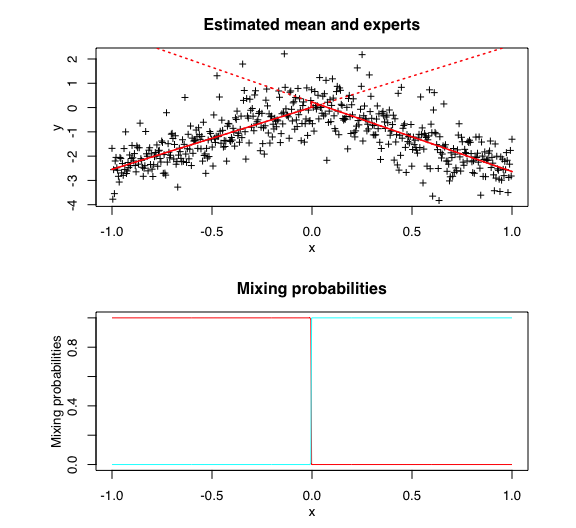### Confidence regions tmoe$plot(what = "confregions")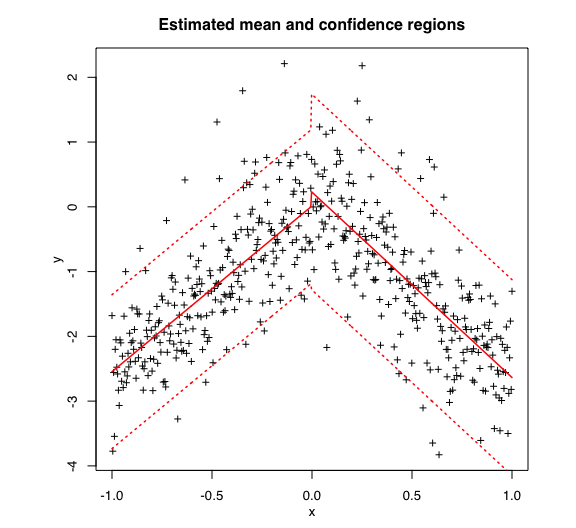tmoe$plot(what = "clusters")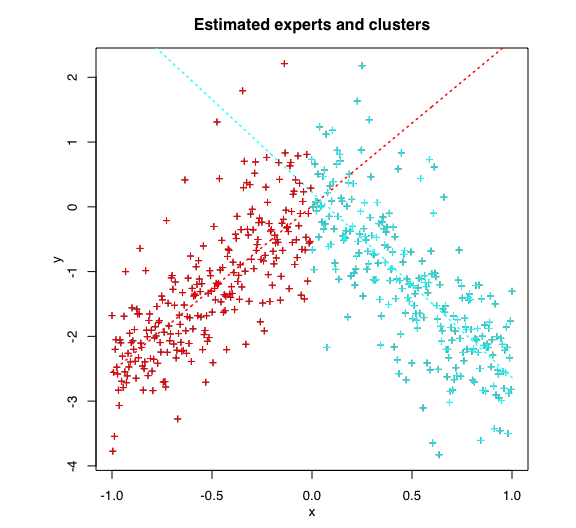### Log-likelihood tmoe$plot(what = "loglikelihood")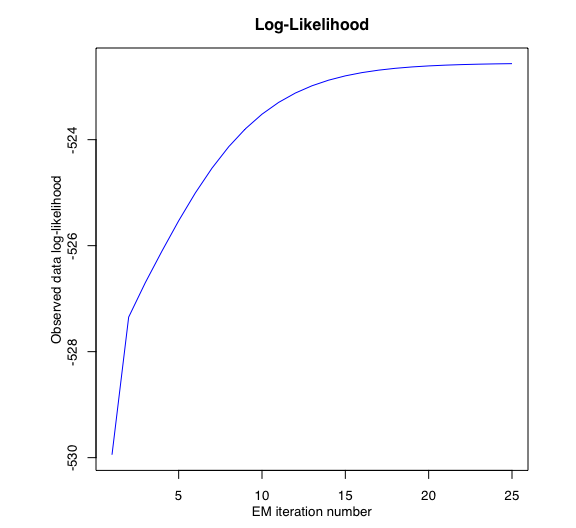# Application to a real dataset

library(MASS)
data("mcycle")
x <- mcycle$times y <- mcycle$accel

## Set up tMoE model parameters

K <- 4 # Number of regressors/experts
p <- 2 # Order of the polynomial regression (regressors/experts)
q <- 1 # Order of the logistic regression (gating network)

## Set up EM parameters

n_tries <- 1
max_iter <- 1500
threshold <- 1e-5
verbose <- TRUE
verbose_IRLS <- FALSE

## Estimation

tmoe <- emTMoE(X = x, Y = y, K, p, q, n_tries, max_iter,
threshold, verbose, verbose_IRLS)
## EM - tMoE: Iteration: 1 | log-likelihood: -607.963023404096
## EM - tMoE: Iteration: 2 | log-likelihood: -603.530462450757
## EM - tMoE: Iteration: 3 | log-likelihood: -600.936924880401
## EM - tMoE: Iteration: 4 | log-likelihood: -597.134488483045
## EM - tMoE: Iteration: 5 | log-likelihood: -587.345068256529
## EM - tMoE: Iteration: 6 | log-likelihood: -579.214908026282
## EM - tMoE: Iteration: 7 | log-likelihood: -575.789415000413
## EM - tMoE: Iteration: 8 | log-likelihood: -574.743067640861
## EM - tMoE: Iteration: 9 | log-likelihood: -573.824355322963
## EM - tMoE: Iteration: 10 | log-likelihood: -572.704434066976
## EM - tMoE: Iteration: 11 | log-likelihood: -571.456957238225
## EM - tMoE: Iteration: 12 | log-likelihood: -570.311351216642
## EM - tMoE: Iteration: 13 | log-likelihood: -569.295154539063
## EM - tMoE: Iteration: 14 | log-likelihood: -568.319884936335
## EM - tMoE: Iteration: 15 | log-likelihood: -567.372601882285
## EM - tMoE: Iteration: 16 | log-likelihood: -566.478212031608
## EM - tMoE: Iteration: 17 | log-likelihood: -565.659310717143
## EM - tMoE: Iteration: 18 | log-likelihood: -564.901676479101
## EM - tMoE: Iteration: 19 | log-likelihood: -564.155447286696
## EM - tMoE: Iteration: 20 | log-likelihood: -563.446620149915
## EM - tMoE: Iteration: 21 | log-likelihood: -562.937110761917
## EM - tMoE: Iteration: 22 | log-likelihood: -562.667086966818
## EM - tMoE: Iteration: 23 | log-likelihood: -562.520110670808
## EM - tMoE: Iteration: 24 | log-likelihood: -562.426801842479
## EM - tMoE: Iteration: 25 | log-likelihood: -562.361727752526
## EM - tMoE: Iteration: 26 | log-likelihood: -562.314424482959
## EM - tMoE: Iteration: 27 | log-likelihood: -562.279516472013
## EM - tMoE: Iteration: 28 | log-likelihood: -562.253254369394
## EM - tMoE: Iteration: 29 | log-likelihood: -562.233295182051
## EM - tMoE: Iteration: 30 | log-likelihood: -562.217975445467
## EM - tMoE: Iteration: 31 | log-likelihood: -562.206116173187
## EM - tMoE: Iteration: 32 | log-likelihood: -562.196865909629
## EM - tMoE: Iteration: 33 | log-likelihood: -562.189597689509
## EM - tMoE: Iteration: 34 | log-likelihood: -562.183858578194
## EM - tMoE: Iteration: 35 | log-likelihood: -562.179299411545

## Summary

tmoe$summary() ## ------------------------------------- ## Fitted t Mixture-of-Experts model ## ------------------------------------- ## ## tMoE model with K = 4 experts: ## ## log-likelihood df AIC BIC ICL ## -562.1793 26 -588.1793 -625.7538 -625.7472 ## ## Clustering table (Number of observations in each expert): ## ## 1 2 3 4 ## 28 37 32 36 ## ## Regression coefficients: ## ## Beta(k = 1) Beta(k = 2) Beta(k = 3) Beta(k = 4) ## 1 -1.0422893 1008.728925 -2132.506787 654.7349946 ## X^1 -0.1089089 -105.713093 135.481456 -27.8267024 ## X^2 -0.0079480 2.481934 -2.112076 0.2888205 ## ## Variances: ## ## Sigma2(k = 1) Sigma2(k = 2) Sigma2(k = 3) Sigma2(k = 4) ## 1.596783 440.1084 473.8641 30.5968 ## Plots ### Mean curve tmoe$plot(what = "meancurve")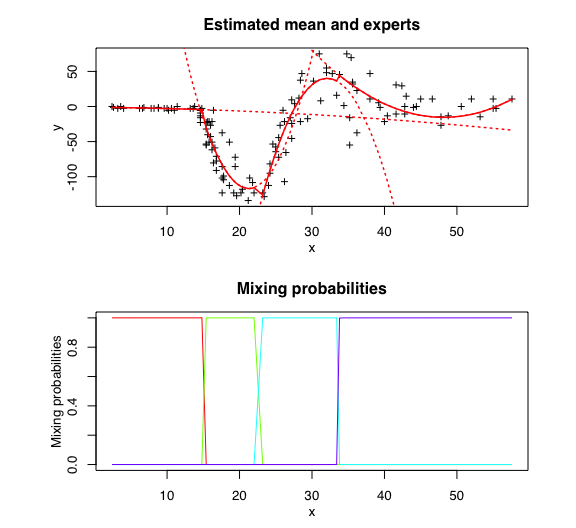tmoe$plot(what = "confregions")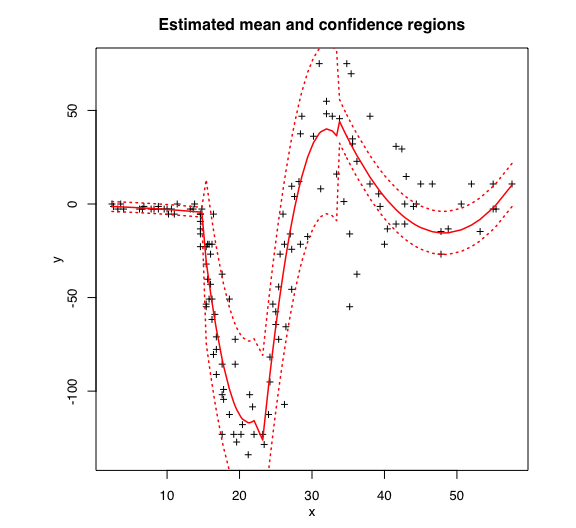### Clusters tmoe$plot(what = "clusters")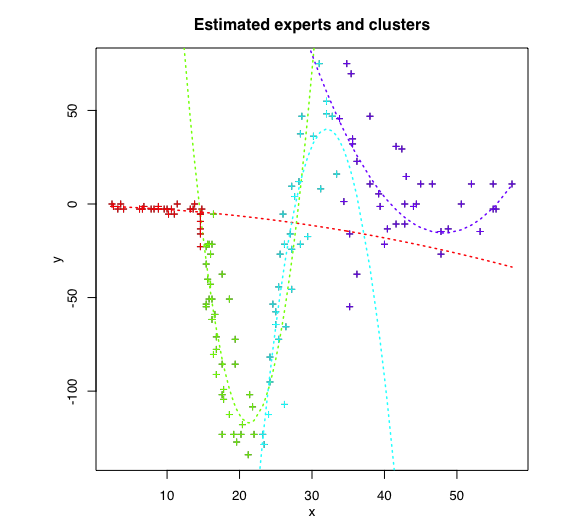### Log-likelihood

tmoe\$plot(what = "loglikelihood")